Now that we know the basics on how to combine signed distance functions, we can use them to do cool stuff with them. In this tutorial we’ll use them to render 2d soft shadows. If you haven’t read my previous tutorials about signed distance fields yet, I highly recommend you do that first, starting at the tutorial about how to create simple shapes.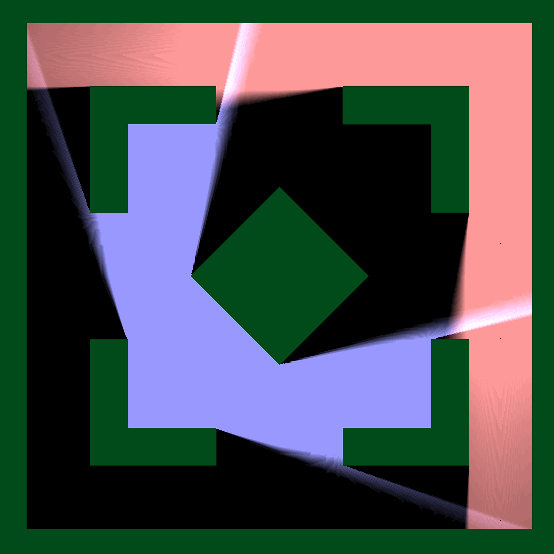## Base Setup

I did a simple room setup here, it uses the techniques described in earlier tutorials. Two things I used that I didn’t explicitely mention previously are that I used a `abs` on a vector2 to mirror the position around both the x and y axis and that I negated a shape distance to flip the inside and outside.

We also copy the 2D_SDF.cginc file from the previous tutorial to the same folder as the shader we’re writing for this one.

``````Shader "Tutorial/037_2D_SDF_Shadows"{
Properties{
}

SubShader{
//the material is completely non-transparent and is rendered at the same time as the other opaque geometry
Tags{ "RenderType"="Opaque" "Queue"="Geometry"}

Pass{
CGPROGRAM

#include "UnityCG.cginc"
#include "2D_SDF.cginc"

#pragma vertex vert
#pragma fragment frag

struct appdata{
float4 vertex : POSITION;
};

struct v2f{
float4 position : SV_POSITION;
float4 worldPos : TEXCOORD0;
};

v2f vert(appdata v){
v2f o;
//calculate the position in clip space to render the object
o.position = UnityObjectToClipPos(v.vertex);
//calculate world position of vertex
o.worldPos = mul(unity_ObjectToWorld, v.vertex);
return o;
}

float scene(float2 position) {
float bounds = -rectangle(position, 2);

float2 quarterPos = abs(position);

float corner = rectangle(translate(quarterPos, 1), 0.5);
corner = subtract(corner, rectangle(position, 1.2));

float diamond = rectangle(rotate(position, 0.125), .5);

float world = merge(bounds, corner);
world = merge(world, diamond);

return world;
}

fixed4 frag(v2f i) : SV_TARGET{
float dist = scene(i.worldPos.xz);
return dist;
}

ENDCG
}
}
FallBack "Standard" //fallback adds a shadow pass so we get shadows on other objects
}
``````

If we still used the visualisation technique we used in the previous tutorials, this shape would look like this: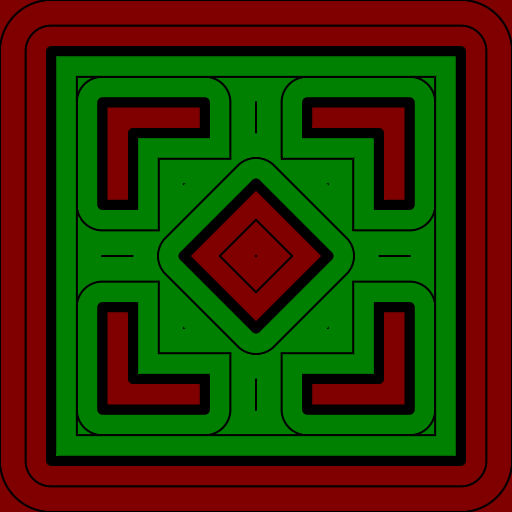## Simple Shadows

For hard shadows, we walk the space from the sample position to he light position. If we find a object on the way there we decide that the pixel should be shadowed and if we get to the light without being interrupted we say that it’s not shadowed.

We start by calculating the base parameters of a ray. We already have the origin (the position of the pixel we’re rendering) and the goal (the position of the light) of the ray. What we need is the length and the normalised direction. We can get the direction by subtracting the start from the destination and normalising the result and the length by subtracting the positions and passing it to the `length` method.

``````float traceShadow(float2 position, float2 lightPosition){
float direction = normalise(lightPosition - position);
float distance = length(lightPosition - position);
}
``````

Then we iterate through the ray in a for loop. We’ll set the iterations of the loop via a define declaration, this allows us to adjust the maximum amount of iterations later and also allows the compiler to optimise the shader a bit by unrolling the loop.

In the loop we need a position at which we currently are, so we declare that outside of the loop with a start value of 0. Then back in the loop we can calculate the sample position by adding the ray progress multiplied by the ray direction to the base position. Then we sample the signed distance function at the position we just calculated.

``````// outside of function
#define SAMPLES 32

// in shadow function
float rayDistance = 0;
for(int i=0 ;i<SAMPLES; i++){
float sceneDist = scene(pos + direction * rayDistance);

//do other stuff and move the ray further
}
``````

Then we can do checks whether we’re already at the point where we can abort the loop. If the scene distance of the signed distance function is near 1, we can assume the ray was blocked by a shape and we return 0. If the ray progress is bigger than the light distance, we can assume that we reached the light without any collisions and return a value of 1.

If we didn’t return yet, we then have to calculate the next sample position. We do that by adding the distance of the scene to the ray progress. The reason for this is that the scene distance gives us the distance to the nearest shape, so if we add that amount to our ray, we can’t possibly cast our ray further than the nearest shape, or even beyond it, which would allow for shadow leaking.

In case we don’t hit anything and also don’t find the light by the time we used our whole sample budget (the loop ended), we also have to return a value. Because this mainly happens near shapes, shortly before the pixel would count as occluded anyways, we’ll use a return value of 0 here.

``````#define SAMPLES 32

float traceShadows(float2 position, float2 lightPosition){
float2 direction = normalize(lightPosition - position);
float lightDistance = length(lightPosition - position);

float rayProgress = 0;
for(int i=0 ;i<SAMPLES; i++){
float sceneDist = scene(position + direction * rayProgress);

if(sceneDist <= 0){
return 0;
}
if(rayProgress > lightDistance){
return 1;
}

rayProgress = rayProgress + sceneDist;
}

return 0;
}
``````

To use the function, we call it in the fragment function with the pixel position and the light position. Then we can multiply the result with any color to tint it in the lights color.

I used the technique described in the first tutorial I made about signed distance fields to also visualise the geometry. Then I simply added the shadows and the geometry. We can use a simple add operation here instead of doing a linear interpolation or similar because the shape color is black everywhere where there’s no shape and the shadow color is black everywhere where there is a shape.

fixed4 frag(v2f i) : SV_TARGET{ float2 position = i.worldPos.xz;

``````float2 lightPos;
sincos(_Time.y, lightPos.x /*sine of time*/, lightPos.y /*cosine of time*/);
float shadows = traceShadows(position, lightPos);
float3 light = shadows * float3(.6, .6, 1);

float sceneDistance = scene(position);
float distanceChange = fwidth(sceneDistance) * 0.5;
float binaryScene = smoothstep(distanceChange, -distanceChange, sceneDistance);
float3 geometry = binaryScene * float3(0, 0.3, 0.1);

float3 col = geometry + light;

return float4(col, 1); }
``````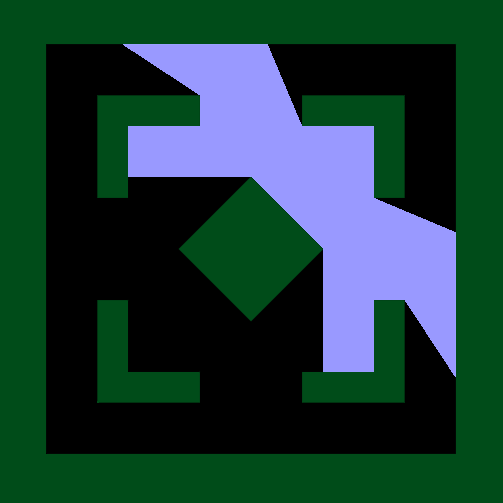## Soft Shadows

The upgrade from those hard shadows to softer, more realistic ones is pretty straightforward and doesn’t make the shader much more performance intensive.

Out first take on this will just get the distance to the nearest scene object for every sample we go through and get the nearest one, then where we previously returned just 1, we can return the distance to the nearest shape. To prevent the shadow intensity to get too high and lead to weird colors when used, we pass it through the `saturate` method which clamps it between 0 and 1. We get the minimum between the current nearest shape and the next one after the check wether the progress reached the light already, otherwise we could take samples that overshot to behind the light and get weird artefacts.

``````float traceShadows(float2 position, float2 lightPosition){
float2 direction = normalize(lightPosition - position);
float lightDistance = length(lightPosition - position);

float rayProgress = 0;
float nearest = 9999;
for(int i=0 ;i<SAMPLES; i++){
float sceneDist = scene(position + direction * rayProgress);

if(sceneDist <= 0){
return 0;
}
if(rayProgress > lightDistance){
return saturate(nearest);
}

nearest = min(nearest, sceneDist);

rayProgress = rayProgress + sceneDist;
}

return 0;
}
``````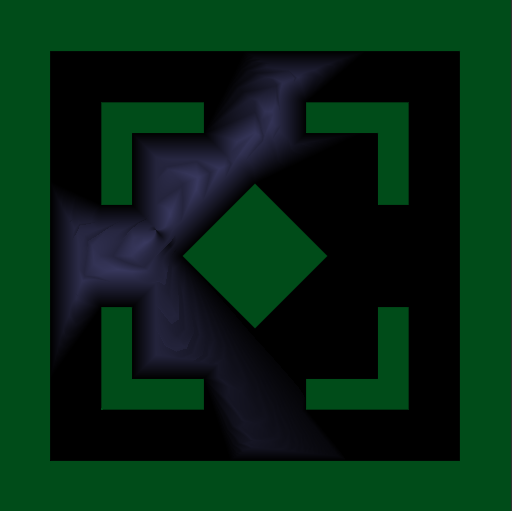The first thing we notice when we do this is the weird “teeth” in the shadows. The reason they appear is that the distance to the scene from the light is less than 1. I tried a lot to counteract that, but didn’t find a solution. What we can do instead is to implement the hardness of the shadows. The hardness will be another parameter in the shadow function. In the loop we multiply the scene distance with the hardness, so with a hardness of 2, the soft, grey part of the shadow is only half as big as before. When using the hardness, the light is allowed to be 1 divided by the hardness close to a shape until the artefacts appear. So if we now use a hardness of 20, we have a distance of 0.05 units we should respect.

``````float traceShadows(float2 position, float2 lightPosition, float hardness){
float2 direction = normalize(lightPosition - position);
float lightDistance = length(lightPosition - position);

float rayProgress = 0;
float nearest = 9999;
for(int i=0 ;i<SAMPLES; i++){
float sceneDist = scene(position + direction * rayProgress);

if(sceneDist <= 0){
return 0;
}
if(rayProgress > lightDistance){
return saturate(nearest);
}

nearest = min(nearest, hardness * sceneDist);

rayProgress = rayProgress + sceneDist;
}

return 0;
}
``````
``````//in fragment function
float shadows = traceShadows(position, lightPos, 20);
``````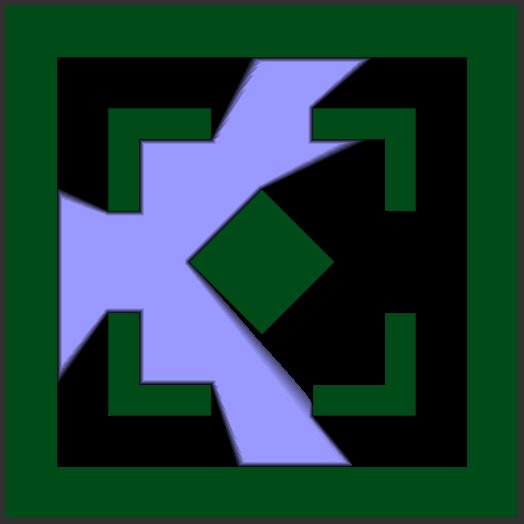With this problem minimised, the next thing we see is that even in the areas that shouldn’t be shadowed, we still see a falloff near walls. Also the softness of the shadow seems similar over the whole shadow, not hard near the shape and softer the further away the point is from the caster.

The way we fix this is by dividing the scene distance by the ray progress. By doing this we divide the distance by very small numbers where the ray starts, that means we still get high numbers and a nice crisp shadow. When we find the nearest point to the ray at a later point on the ray, the nearest point gets divided by a bigger number, making the shadow softer. Because this doesn’t have that much to do with the nearest distance, we’ll also rename the variable to `shadow`.

Another small change we’ll make is that because we’re dividing by rayProgress, starting at 0 is a bad idea (dividing by zero is almost always a bad idea). Any very small number is fine as a start here.

``````float traceShadows(float2 position, float2 lightPosition, float hardness){
float2 direction = normalize(lightPosition - position);
float lightDistance = length(lightPosition - position);

float rayProgress = 0.0001;
float shadow = 9999;
for(int i=0 ;i<SAMPLES; i++){
float sceneDist = scene(position + direction * rayProgress);

if(sceneDist <= 0){
return 0;
}
if(rayProgress > lightDistance){
return saturate(shadow);
}

shadow = min(shadow, hardness * sceneDist / rayProgress);

rayProgress = rayProgress + sceneDist;
}

return 0;
}
``````## Multiple lights

In this simple one-shader implementation the easiest way to get multiple lights is to calculate them separately and then add all of the results.

``````fixed4 frag(v2f i) : SV_TARGET{
float2 position = i.worldPos.xz;

float2 lightPos1 = float2(sin(_Time.y), -1);
float shadows1 = traceShadows(position, lightPos1, 20);
float3 light1 = shadows1 * float3(.6, .6, 1);

float2 lightPos2 = float2(-sin(_Time.y) * 1.75, 1.75);
float shadows2 = traceShadows(position, lightPos2, 10);
float3 light2 = shadows2 * float3(1, .6, .6);

float sceneDistance = scene(position);
float distanceChange = fwidth(sceneDistance) * 0.5;
float binaryScene = smoothstep(distanceChange, -distanceChange, sceneDistance);
float3 geometry = binaryScene * float3(0, 0.3, 0.1);

float3 col = geometry + light1 + light2;

return float4(col, 1);
}
``````## Source

### 2D Soft Shadows

``````Shader "Tutorial/037_2D_SDF_Shadows"{
Properties{
}

SubShader{
//the material is completely non-transparent and is rendered at the same time as the other opaque geometry
Tags{ "RenderType"="Opaque" "Queue"="Geometry"}

Pass{
CGPROGRAM

#include "UnityCG.cginc"
#include "2D_SDF.cginc"

#pragma vertex vert
#pragma fragment frag

struct appdata{
float4 vertex : POSITION;
};

struct v2f{
float4 position : SV_POSITION;
float4 worldPos : TEXCOORD0;
};

v2f vert(appdata v){
v2f o;
//calculate the position in clip space to render the object
o.position = UnityObjectToClipPos(v.vertex);
//calculate world position of vertex
o.worldPos = mul(unity_ObjectToWorld, v.vertex);
return o;
}

float scene(float2 position) {
float bounds = -rectangle(position, 2);

float2 quarterPos = abs(position);

float corner = rectangle(translate(quarterPos, 1), 0.5);
corner = subtract(corner, rectangle(position, 1.2));

float diamond = rectangle(rotate(position, 0.125), .5);

float world = merge(bounds, corner);
world = merge(world, diamond);

return world;
}

#define STARTDISTANCE 0.00001
#define MINSTEPDIST 0.02
#define SAMPLES 32

float traceShadows(float2 position, float2 lightPosition, float hardness){
float2 direction = normalize(lightPosition - position);
float lightDistance = length(lightPosition - position);

float lightSceneDistance = scene(lightPosition) * 0.8;

float rayProgress = 0.0001;
float shadow = 9999;
for(int i=0 ;i<SAMPLES; i++){
float sceneDist = scene(position + direction * rayProgress);

if(sceneDist <= 0){
return 0;
}
if(rayProgress > lightDistance){
return saturate(shadow);
}

shadow = min(shadow, hardness * sceneDist / rayProgress);
rayProgress = rayProgress + max(sceneDist, 0.02);
}

return 0;
}

fixed4 frag(v2f i) : SV_TARGET{
float2 position = i.worldPos.xz;

float2 lightPos1 = float2(sin(_Time.y), -1);
float shadows1 = traceShadows(position, lightPos1, 20);
float3 light1 = shadows1 * float3(.6, .6, 1);

float2 lightPos2 = float2(-sin(_Time.y) * 1.75, 1.75);
float shadows2 = traceShadows(position, lightPos2, 10);
float3 light2 = shadows2 * float3(1, .6, .6);

float sceneDistance = scene(position);
float distanceChange = fwidth(sceneDistance) * 0.5;
float binaryScene = smoothstep(distanceChange, -distanceChange, sceneDistance);
float3 geometry = binaryScene * float3(0, 0.3, 0.1);

float3 col = geometry + light1 + light2;

return float4(col, 1);
}

ENDCG
}
}
FallBack "Standard"
}
``````

This is only one of many uses of signed distance fields. So far they’re a bit unweildy because all shapes have to be hardcoded into a shader or passed via shader properties, but I have a few ideas on how to make them more usable with future tutorials.

You can also find me on twitter at @totallyRonja. If you liked my tutorial and want to support me you can do that on Patreon (patreon.com/RonjaTutorials) or Ko-Fi (ko-fi.com/RonjaTutorials).